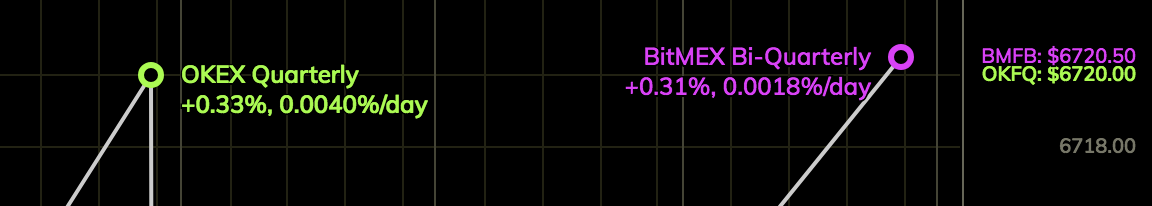July 9, 2018

The addition of the Futures curve to Clark Moody Bitcoin was the first real-time futures visualization in the Bitcoin space.

Today, Futures gets a small but important upgrade with the addition of a live label on the chart. Below the market, I’ve added two percentage values: the premium over spot and the implied growth rate.The first percentage is simply the contract premium (or discount) over the index underlying that contract. BitMEX and OKEX maintain separate indices for settlement of their respective futures contracts.

``````Premium = 100 * (Contract Price / Index Price - 1)
``````

## Implied Growth Rate

The second number is the implied daily growth rate. Given the contract premium over the index and the number of days remaining until contract expiration, the implied growth rate is the daily compound return needed to arrive at the contract price.

``````Implied = 100 * ((Contract Price / Index Price)^(1 / Days) - 1)
``````

## Feedback

As always, please let me know how I can make the Futures page better.

Visit Futures Now